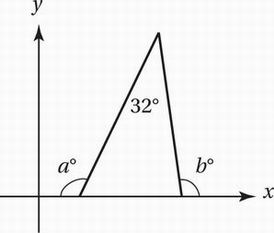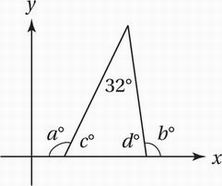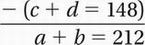# SAT Math Multiple Choice Question 757: Answer and Explanation

### Test Information

Question: 757

7.In the figure above, what is the value of a + b?

• A. 212
• B. 238
• C. 296
• D. 328

Explanation:

A

It helps to mark the measures of the other two interior angles to the triangle as c° and d°, because we know something about these angles.Since angles in a triangle must have a sum of 180°:

c + d + 32 = 180

Subtract 32:

c + d = 148

Since angles in a linear pair have a sum of 180°:

a + c = 180

b + d = 180